Example Questions

Example Question #61 : Basic Squaring / Square Roots

If (x - 3)2 = 64, what is a possible value of x?

10
8
5
-8
-5
Explanation:

In order to solve for x, we must first take the square root of both sides.

(x - 3)2 = 64

(x - 3) = +/- 8

The square root of 64 is either 8 or -8. We then solve the equation with both possible values of the square root of 64.

x - 3 = 8 (Add 3 to both sides.)

x = 11

x - 3 = -8 (Add 3 to both sides.)

x = -5

x = 11 or -5. -5 is the only possible value of x that is an answer choice.

Example Question #1154 : Act Math

Solve: 3³

93

9

99

27

18

27

Explanation:

When using a cube root. 3³ becomes 3 * 3 * 3.

3 * 3 = 9

9 * 3 = 27

Example Question #1155 : Act Math

When you square the following numbers, which one results in an irrational number?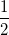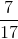Explanation:

A rational number is any number than can be expressed as a fraction or a quotient of two integers. The square of π is still an irrational number. (√(2))2 = 2, (1/3)2 = 1/9, (7/17)2 = 49/289, (1/2)2 = 1/4, all can be writen in fractional form, and are thus, rational numbers.

Example Question #1 : Other Squaring / Square Roots

How much larger is the sum of the squares of –2, –3, and 4 than the sum of these integers?

Infinitely larger

17

25

30

–1

30

Explanation:

The square of –2 is 4, of –3 is 9, and of 4 is 16.

The sum of these squares is 29.

The sum of (–2) + (–3) + (4) is –1.

29 – (–1) = 30

Example Question #1157 : Act Math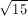is between what two integers?andandandandandandExplanation: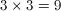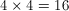falls betweenandTherefore,is betweenandExample Question #1158 : Act Math

Simplify the following: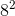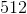Explanation:is the same asmultiplied by. Thus, the answer is.

Example Question #1 : How To Find The Square Of An Integer

The last two digits in the square of a numberhave a product of. Which of the following could NOT be the last digit of?If the last two digits of the squared number multiply to, then the digits must be factors of. The last digit of any square of a number ending inwill be, which is not a factor of. Thus, the number cannot end in.